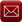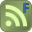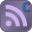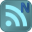HomeShowcaseMaking Excel Read Graphical and Tabular Data
 Making Excel Read Graphical and Tabular Data

When you add a trendline to a chart, Excel provides an option to display the trendline equation in the chart. This tip describes how to create formulas that generate the trendline coefficients. You can then use these formulas to calculate predicted y values for give values of x.

1) First the Easy Way

These videos are best viewed in full screen mode. Press play and double click the screen to maximise.

2) Now the More Complex 'Dynamic' Way

These videos are best viewed in full screen mode. Press play and double click the screen to maximise.

The following cell formulae will dynamically calculate trendline equation parameters from a data table. The trendline formulae are quite complicated so copy and paste into your worksheet. These equations assume that your sheet contains C5:H5 (known_x's) and C8:H8 (known_y's).

Linear Trendline

Equation: y = m * x + b
m: =SLOPE(C8:H8,C5:H5)
b: =INTERCEPT(C8:H8,C5:H5)

Logarithmic Trendline
Equation: y = (c * LN(x)) + b
c: =INDEX(LINEST(C8:H8,LN(C5:H5)),1)
b: =INDEX(LINEST(C8:H8,LN(C5:H5)),1,2)

Power Trendline
Equation: y=c*x^b
c: =EXP(INDEX(LINEST(LN(C8:H8),LN(C5:H5),,),1,2))
b: =INDEX(LINEST(LN(C8:H8),LN(C5:H5),,),1)

Exponential Trendline
Equation: y = c *e ^(b * x)
c: =EC5:H5P(INDEX(LINEST(LN(C8:H8),C5:H5),1,2))
b: =INDEX(LINEST(LN(C8:H8),C5:H5),1)

2nd Order Polynomial Trendline
Equation: y = (c2 * x^2) + (c1 * x ^1) + b
c2: =INDEX(LINEST(C8:H8,C5:H5^{1,2}),1)
C1: =INDEX(LINEST(C8:H8,C5:H5^{1,2}),1,2)
b = =INDEX(LINEST(C8:H8,C5:H5^{1,2}),1,3)

3rd Order Polynomial Trendline
Equation: y = (c3 * x^3) + (c2 * x^2) + (c1 * x^1) + b
c3: =INDEX(LINEST(C8:H8,C5:H5^{1,2,3}),1)
c2: =INDEX(LINEST(C8:H8,C5:H5^{1,2,3}),1,2)
C1: =INDEX(LINEST(C8:H8,C5:H5^{1,2,3}),1,3)
b: =INDEX(LINEST(C8:H8,C5:H5^{1,2,3}),1,4)

Higher Order Polynomial Trendline
Follow the pattern set in the second and third order polynomial trendlines.

In the Forum

Some Sample Calculations on the SitePurpose of calculation: Calculate stress in a 'semi circular' tension fitting (or bath tub fitting).

###Angle type tension fitting.xlsPurpose of calculation: Calculate stress in a 'angle' tension fitting...

###Channel type tension fitting.xlsPurpose of calculation: Calculate stress in a 'channel' tension fitting...

We have 13 guests and 37 members onlineEmail (preferred method of contact)US +1 617 5008224EU +44 113 8152220